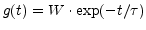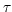StaticSpikingCbSynapse
Subsections

## StaticSpikingCbSynapse

We call a synapse a static synapse if the amplitude of each evoked conductance change is equal. Here we implement a conductance g(t) of the formfor each spike which hits the synapse at time t with an amplitude of W and a decay time constant of. The responses of all the spikes are added up linearly.

E :
Equilibrium potential given by the Nernst equation.
tau (sec) :
The synaptic time constantW :
The weight (scaling factor, strenght, maximal amplitude) of the synapse
delay (sec) :
The synaptic transmission delay